# All you need to know about Inverse Document Frequency

Published on 6th Mar 2020

TF-IDF is one of the most popular measures that quantify document relevance for a given term. It is extensively used in Information Retrieval (ex: Search Engines), Text Mining and even for text-heavy Machine Learning use cases like Document Classification and Clustering. Today we explore the better half of TF-IDF and see its connection with Probability, the role it plays in TF-IDF and even the intuition behind it.

Inverse Document Frequency (IDF) is a measure of term rarity which means it quantifies how rare the term, in the corpus, really is (document collection); higher the IDF, rarer the term. A rare term helps in discriminating, distinguishing and ranking documents and it contributes more information to the corpus than what a more frequent term (like `a`, `and` and `the`) does.

The IDF was heuristically proposed in the paper "A statistical interpretation of term specificity and its application in retrieval" (Spärck Jones, 1972) and was originally called Term Specificity.

# The intuition behind IDF

In order to quantify the term rarity, the heuristic says we need to give higher weight to the term that occurs in fewer documents and lesser weights to the frequent ones. Thus this measure (weight) `w` of the term is inversely proportional to the number of documents in which it is present (called Document Frequency) - and hence the measure is called Inverse Document Frequency.Any function that adheres to the requirement of being inversely proportional to the document frequency i.e. a decreasing function, would do the job; it may not yield optimality but could be used as an IDF for some use cases. Some decreasing functions that could be used as an IDF for some use cases are shown below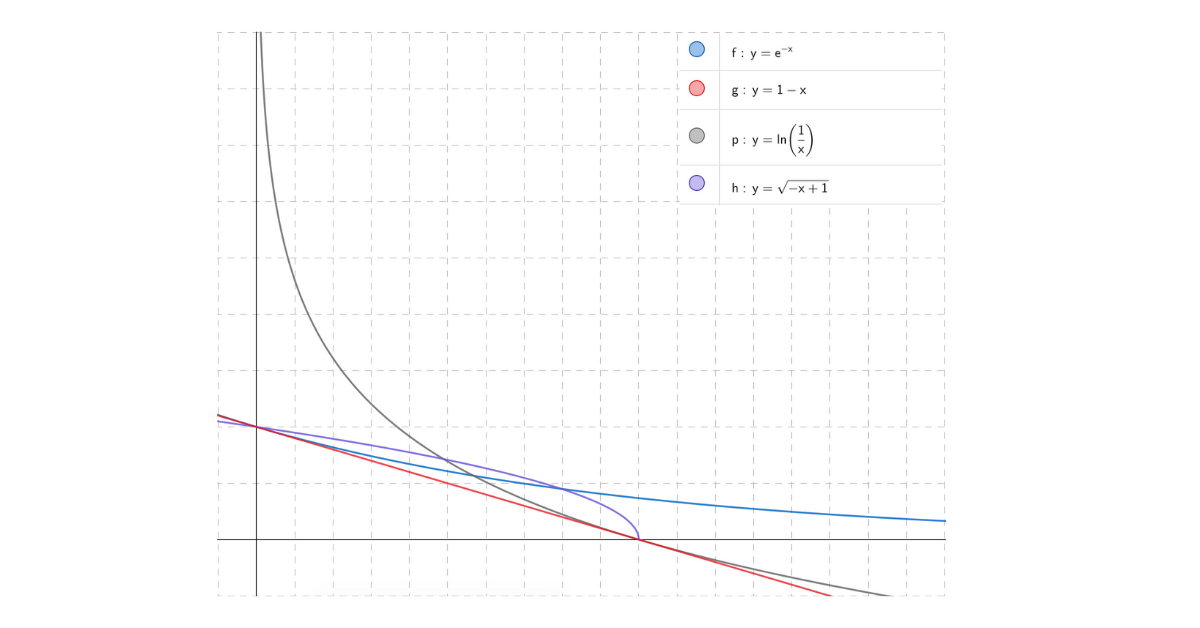The more frequent words, like `a`, `and` and `the` will lie on the far right of the plot and will have a smaller value of IDF.

# The most common IDF

A widely adapted IDF measure that performs better in most use cases is defined belowwhere

• `N` is the number of documents in the corpus
• `df(t)` is the number of documents that has an occurrence of the term `t`

If we plot the above IDF function against the document frequency we get a nice smooth decreasing function as shown below. For lower values of X i.e. Document Frequency we see the IDF is very high as it suggests a good discriminator and as the Document Frequency increases the plot smoothly descends and reaches 0 for `df(t) = N`.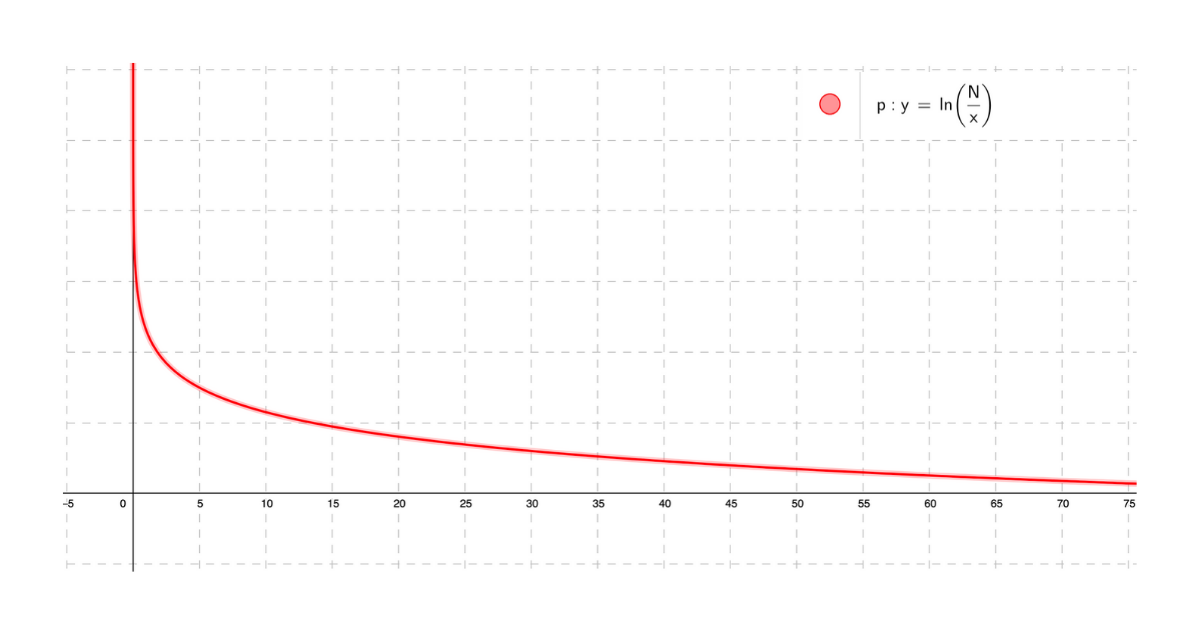# IDF and Probability

What would be the probability that a random document picked from a corpus of `N` documents contains the term `t`? The answer to this question is the fraction of documents, out of N, that contains the term `t` and, as seen above, this is its Document Frequency.The fraction inside the logarithm in the IDF function is oddly similar to the above probability, in fact, it is the inverse of probability defined above. Hence we could redefine IDF using this probability asBy defining IDF as a probability, we could now estimate the true IDF of a term by observing a random sample instead and computing IDF on this sampled data.

# IDF of conjunction

Computing IDF for a single term is fine but what happens when we have multiple terms? How would that fare out? This is a very common use case in Information Retrieval where we need to rank documents for a given search query, and the search query more often than not contains multiple terms.

For finding IDF of multiple terms in conjunction we make an assumption - the occurrences of terms are statistically independent and because of this the equation below holds trueGiven this, we could derive the IDF of two terms in conjunction as follows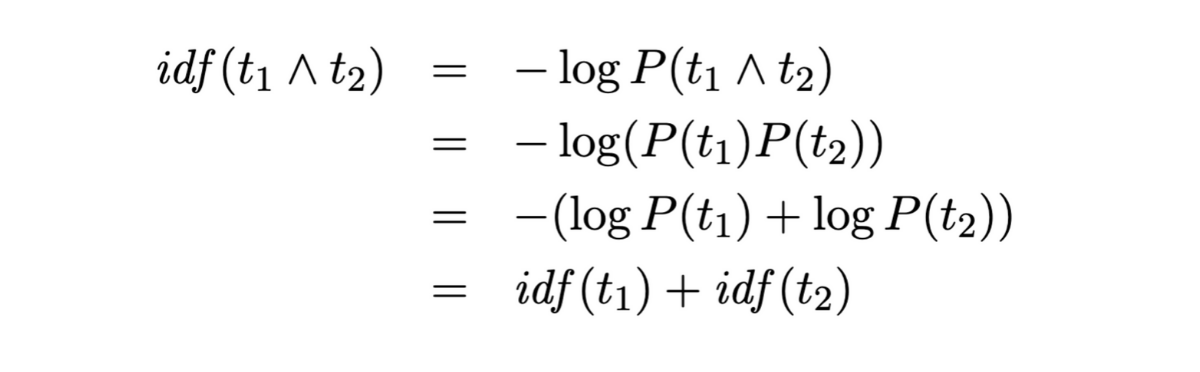From the derivation above we see that the IDF of conjunction is just the summation of IDF of individual terms. Extending this to search engines we could say that the score of a document for a given search query is the summation of scores that document gets for individual terms of the query.

Note: IDF on conjunction could be made much more complex by not assuming statistical independence.

# Other measures of IDF

The decreasing functions we see in the first section of this article were just some examples of possible IDF functions. But there are IDF functions that are not just examples but are also used in some specific use cases and some of them are: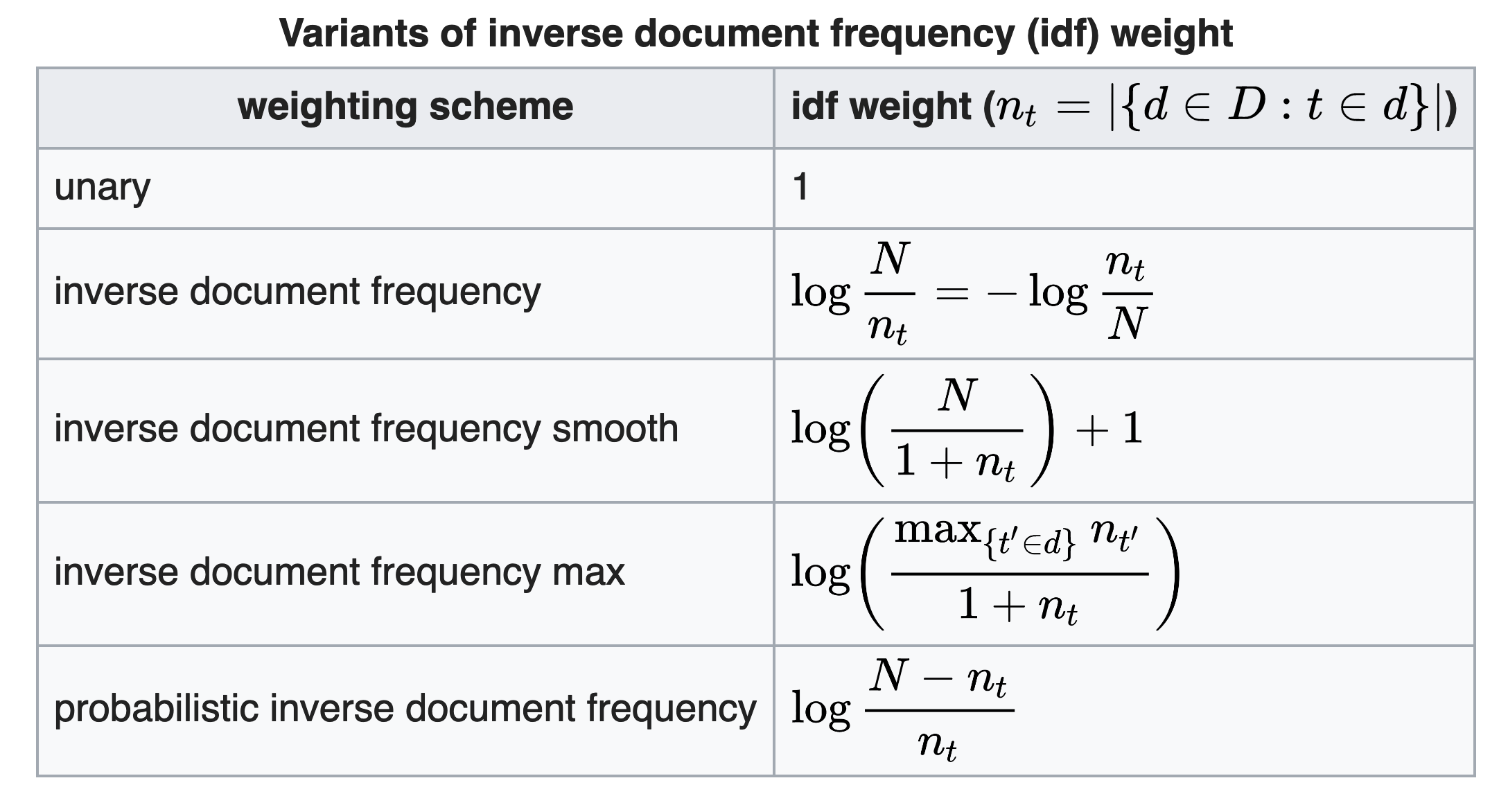Most of the IDF functions only differ in the bounds they produce for a given range of document frequency. The plots of 3 IDF functions namely - Common IDF, Smooth IDF, and Probabilistic IDF, are shown below: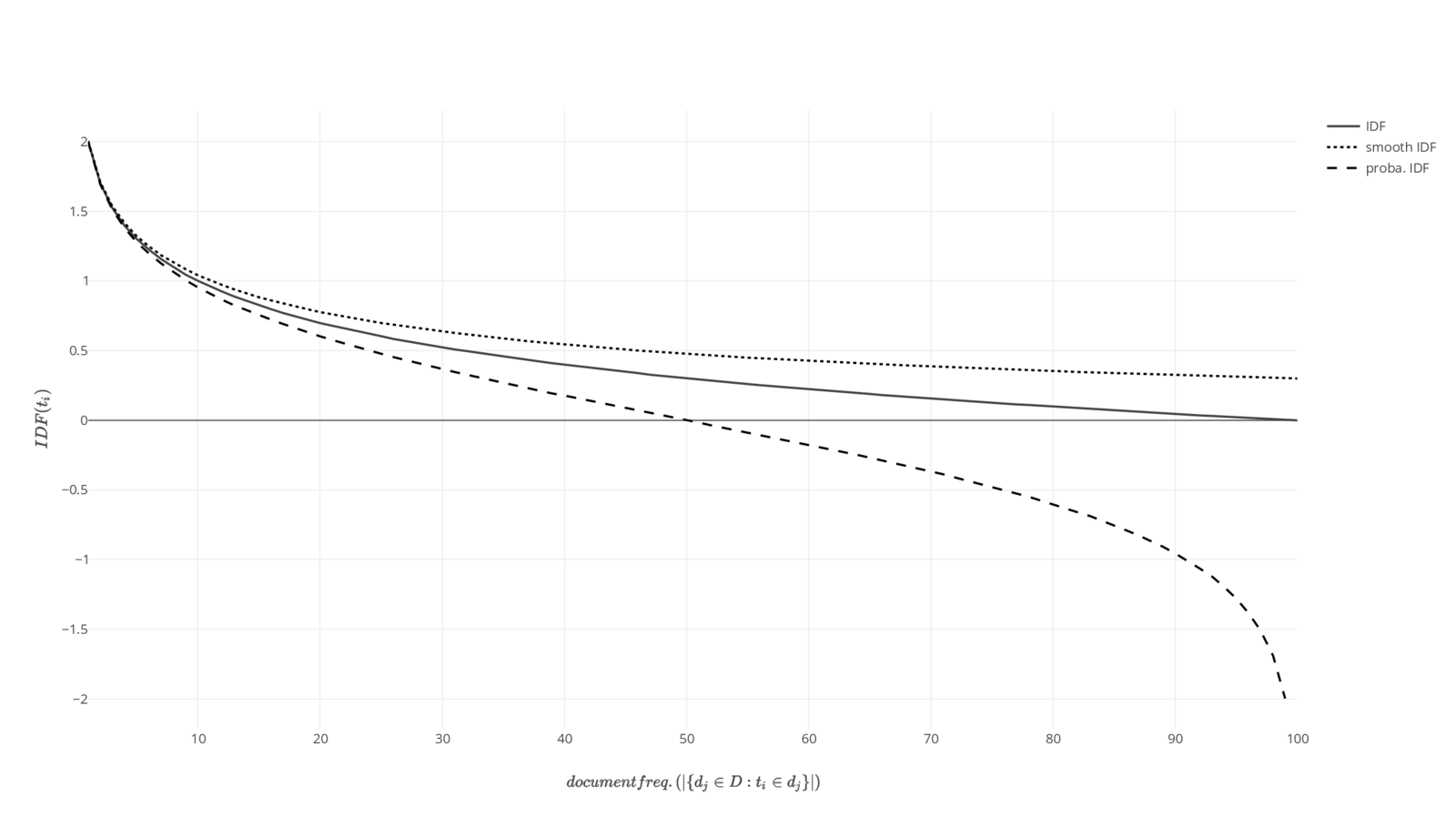By observing the plots of 3 different IDF functions it becomes clear that we should use Probabilistic IDF function when we want to penalize a term that occurs in more than 50% of document by giving it a negative weight; and use a Smooth IDF when we do not want a bounded IDF value and not `undefined` (for `DF(t) = 0`) and `0` (for `DF(t) = N`) as such values ruins a function where IDF is multiplied with some other scalar (like Term Frequency).

Similarly, we could define our own IDF function by deciding when and how the penalty to be applied and defining the parameters accordingly.

# Role of IDF in TF-IDF

TF-IDF suggests how important a word is to a document in a collection (corpus). It helps search engines identify what it is that makes a given document special for a given query. It is defined as the product of Term Frequency (number of occurrences of the term in the document) and Inverse Document Frequency.

For the document to have a high TF-IDF score (high relevance) it needs to have high term frequency and a high inverse document frequency (i.e. low document frequency) of the term. Thus IDF primarily downscales the frequent occurring of common words and boosts the infrequent words with high term frequency.

# References

Other references:

Images used in other measures of IDF are taken from Wikipedia page of TF-IDF.## 500+ Signups

If you like what you read subscribe you can always subscribe to my newsletter and get the post delivered straight to your inbox. I write essays on various engineering topics and share it through my weekly newsletter 👇

# Other articles that you might likeSolving an age-old problem using Bayesian Average

Ranking a list of movies, products, books or even restaurants is tricky and in this article, we find...

12th AprPowering inheritance in C using structure composition

C language does not support inheritance however it does support Structure Compositions which can be ...

7th JunI changed my Python and made it dubious | Python Internals